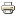# Policy convergence in a two-candidate probabilistic voting modelArticle
Author/s:
Alexei V. Zakharov, Constantine S. Sorokin
Social Choice and Welfare
Issue number:
August 2014, Volume 43, Issue 2,
Year:
2014
Journal pages:
pp 429-446
We propose a generalization of the probabilistic voting model in two-candidate elections. We allow the candidates have general von Neumann–Morgenstern utility functions defined over the voting outcomes. We show that the candidates will choose identical policy positions only if the electoral competition game is constant-sum, such as when both candidates are probability-of-win maximizers or vote share maximizers, or for a small set of functions that for each voter define the probability of voting for each candidate, given candidate policy positions. At the same time, a pure-strategy local Nash equilibrium (in which the candidates do not necessarily choose identical positions) exists for a large set of such functions. Hence, if the candidate payoffs are unrestricted, the “mean voter theorem” for probabilistic voting models is shown to hold only for a small set of probability of vote functions.
Developed by Paolo Gittoi